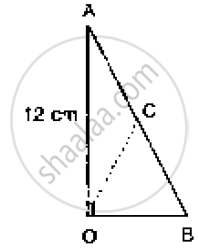# In the Below Fig. ∠Aob = 90°, Ac = Bc, Oa = 12 Cm and Oc = 6.5 Cm. Find the Area of δAob. - Mathematics

In the below fig. ∠AOB = 90°, AC = BC, OA = 12 cm and OC = 6.5 cm. Find the area of
ΔAOB.#### Solution

Since, the midpoint of the hypotenuse of a right triangle is equidistant from the vertices

∴ CA = CB = OC

⇒ AB = 13 = 6.5cm

In a right angle triangle OAB, we have

AB2 = OB2 + OA2

⇒ 132 = OB2 + 122

⇒ OB2 = 132 - 122 = 169 - 144 = 25

⇒ OB = 5

∵ ar (ΔAOB) = 1/2 (OA×OB) = 1/2 (12 ×5 ) = 30cm2

Concept: Concept of Area
Is there an error in this question or solution?
Chapter 14: Areas of Parallelograms and Triangles - Exercise 14.3 [Page 44]

#### APPEARS IN

RD Sharma Mathematics for Class 9
Chapter 14 Areas of Parallelograms and Triangles
Exercise 14.3 | Q 4 | Page 44
Share